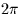Next: SLA_CS2C - Spherical to Cartesian
Up: SUBPROGRAM SPECIFICATIONS
Previous: SLA_CR2AF - Radians to Deg,Arcmin,Arcsec

## SLA_CR2TF - Radians to Hour,Min,Sec

ACTION:
Convert an angle in radians to hours, minutes, seconds (single precision).

CALL:
CALL sla_CR2TF (NDP, ANGLE, SIGN, IHMSF)

GIVEN:

 I NDP number of decimal places of seconds ANGLE R angle in radians

RETURNED:

 C SIGN +' or -' IHMSF I(4) hours, minutes, seconds, fraction

NOTES:
1.
NDP less than zero is interpreted as zero.
2.
The largest useful value for NDP is determined by the size of ANGLE, the format of REAL floating-point numbers on the target machine, and the risk of overflowing IHMSF(4). For example, on a VAX computer, for ANGLE up to, the available floating-point precision corresponds roughly to NDP=3. This is well below the ultimate limit of NDP=9 set by the capacity of the 32-bit integer IHMSF(4).
3.
The absolute value of ANGLE may exceed. In cases where it does not, it is up to the caller to test for and handle the case where ANGLE is very nearlyand rounds up to 24 hours, by testing for IHMSF(1)=24 and setting IHMSF(1-4) to zero.Next: SLA_CS2C - Spherical to Cartesian
Up: SUBPROGRAM SPECIFICATIONS
Previous: SLA_CR2AF - Radians to Deg,Arcmin,Arcsec

SLALIB --- Positional Astronomy Library